Home

# Breaking-onset, energy and momentum flux in unsteady focused wave packets

## Abstract

Breaking waves on the ocean surface transfer energy and momentum into currents and turbulence. What is less well understood, however, is the associated total loss of wave energy and momentum flux. Further, finding a robust and universal diagnostic parameter that determines the onset of breaking and its strength is still an open question. Derakhti & Kirby (J. Fluid Mech., vol. 761, 2014, pp. 464–506) have recently studied bubble entrainment and turbulence modulation by dispersed bubbles in isolated unsteady breaking waves using large-eddy simulation. In this paper, a new diagnostic parameter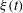${\it\xi}(t)$ is defined based on that originally proposed by Song & Banner (J. Phys. Oceanogr., vol. 32, 2002, pp. 2541–2558), and it is shown that using a threshold value of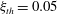${\it\xi}_{th}=0.05$ , the new dynamic criteria is capable of detecting single and multiple breaking events in the considered packets. In addition, the spatial variation of the total energy and momentum flux in intermediate- and deep-water unsteady breaking waves generated by dispersive focusing is investigated. The accuracy of estimating these integral measures based on free surface measurements and using a characteristic wave group velocity is addressed. It is found that the new diagnostic parameter just before breaking,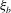${\it\xi}_{b}$ , has a strong linear correlation with the commonly used breaking strength parameter$b$ , suggesting that${\it\xi}_{b}$ can be used to parameterize the averaged breaking-induced dissipation rate and its associated energy flux loss. It is found that the global wave packet time and length scales based on the spectrally weighted packet frequency proposed by Tian et al. (J. Fluid Mech., vol. 655, 2010, pp. 217–257), are the reasonable estimations of the time and length scales of the carrier wave in the packet close to the focal/break point. A global wave steepness,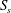$S_{s}$ , is defined based on these spectrally weighted scales, and its spatial variation across the breaking region is examined. It is shown that the corresponding values of$S_{s}$ far upstream of breaking,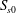$S_{s0}$ , have a strong linear correlation with respect to$b$ for the considered focused wave packets. The linear relation, however, cannot provide accurate estimations of$b$ in the range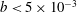$b<5\times 10^{-3}$ . A new scaling law given by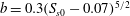$b=0.3(S_{s0}-0.07)^{5/2}$ , which is consistent with inertial wave dissipation scaling of Drazen et al. (J. Fluid Mech., vol. 611, 2008, pp. 307–332), is shown to be capable of providing accurate estimates of$b$ in the full range of breaking intensities, where the scatter of data in the new formulation is significantly decreased compared with that proposed by Romero et al. (J. Phys. Oceanogr., vol. 42, 2012, pp. 1421–1444). Furthermore, we examine nonlinear interactions of different components in a focused wave packet, noting interactive effect on a characteristic wave group velocity in both non-breaking and breaking packets. Phase locking between spectral components is observed in the breaking region as well, and subsequently illustrated by calculating the wavelet bispectrum.

## References

Hide All
MathJax
MathJax is a JavaScript display engine for mathematics. For more information see http://www.mathjax.org.

# Breaking-onset, energy and momentum flux in unsteady focused wave packets

## Metrics

### Full text viewsFull text views reflects the number of PDF downloads, PDFs sent to Google Drive, Dropbox and Kindle and HTML full text views.

Total number of HTML views: 0
Total number of PDF views: 0 *Loading metrics...

### Abstract viewsAbstract views reflect the number of visits to the article landing page.

Total abstract views: 0 *Loading metrics...

* Views captured on Cambridge Core between <date>. This data will be updated every 24 hours.

Usage data cannot currently be displayed